# Three numbers

Find three numbers so that the second number is 4 times greater than the first and the third is lower by 5 than the second number. Their sum is 67.

Correct result:

a =  8
b =  32
c =  27

#### Solution:

b = 4a
c = b - 5
a+b+c=67

b = 4•a
c = b - 5
a+b+c=67

4a-b = 0
b-c = 5
a+b+c = 67

a = 8
b = 32
c = 27

Calculated by our linear equations calculator.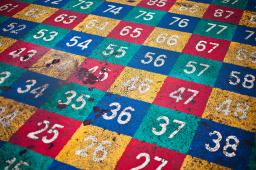We would be very happy if you find an error in the example, spelling mistakes, or inaccuracies, and please send it to us. We thank you!Tips to related online calculators
Do you have a system of equations and looking for calculator system of linear equations?

## Next similar math problems:

• Part-time workersThree part-time workers received CZK 1,235 for their work. The first received 20% less than the second and the third received 45 CZK more than the second. Determine how many crowns (CZK) each of them received.
• The agesThe ages of the four sons make an arithmetic sequence, the sum of which is the age of the father today. In three years, the father's age will be the sum of the ages of the three eldest sons, and in the next two years and three months, the father's age wil
• Kohlrabies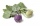The price of one kohlrabi increased by € 0.40. The number of kohlrabies that a customer can buy for € 4 has thus decreased by 5. Find out the new price of one kohlrabi in euros.
• Dimensions of the trapezoidOne of the bases of the trapezoid is one-fifth larger than its height, the second base is 1 cm larger than its height. Find the dimensions of the trapezoid if its area is 115 cm2
• Find d 2Find d in an A. P. whose 5th term is 18 and 39th term is 120.
• Find the 13Find the equation of the circle inscribed in the rhombus ABCD where A[1, -2], B[8, -3] and C[9, 4].
• Two teas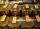A mixture weighing 10 kg was made from two types of tea. The price of tea was 160 CZK/kg and second tea 170 CZK/kg. The price of the mixture is 166 CZK/kg. How many kilograms of each type of tea had to be mixed?
• Father and son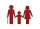When I was eight years old, my father was 30 years old. Today, my father is 3 times more than me. How old am I?
• Journey to spaMr. Dvorak took his wife to the spa and the journey took 3.5 hours. On the way back, he drove 12 km/h faster and was home in 3 hours. At what speed did he go to the spa and at what speed did he return?
• When IWhen I am as old as my older brother is today, my brother will be twice as old as I am today. a) How old is my brother if I am 8 years old today? b) How old am I if my brother is 21 today?
• Railway embankmentThe section of the railway embankment is an isosceles trapezoid, the sizes of the bases of which are in the ratio 5: 3. The arms have a length of 5 m and the height of the embankment is 4.8 m. Calculates the size of the embankment section area.
• Area and perimeter of rectangleThe content area of the rectangle is 3000 cm2, one dimension is 10 cm larger than the other. Determine the perimeter of the rectangle.
• There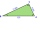There is a triangle ABC: A (-2,3), B (4, -1), C (2,5). Determine the general equations of the lines on which they lie: a) AB side, b) height to side c, c) Axis of the AB side, d) median ta to side a
• Isosceles triangleIn an isosceles triangle ABC with base AB; A [3,4]; B [1,6] and the vertex C lies on the line 5x - 6y - 16 = 0. Calculate the coordinates of vertex C.
• Soccer balls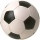Pupils in one class want to buy two soccer balls together. If each of them brings 12.50 euros, they will miss 100 euros, if each brings 16 euros, they will remain 12 euros. How many students are in the class?
• The water barrel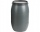The water barrel weighs 122 kg. If we pour 75% of the water out of it, it will weigh 35 kg. What is the weight of the barrel?
• Line intersect segment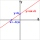Decide whether the line p : x + 2 y - 7 = 0 intersects the line segment given by points A[1, 1] and B[5, 3]